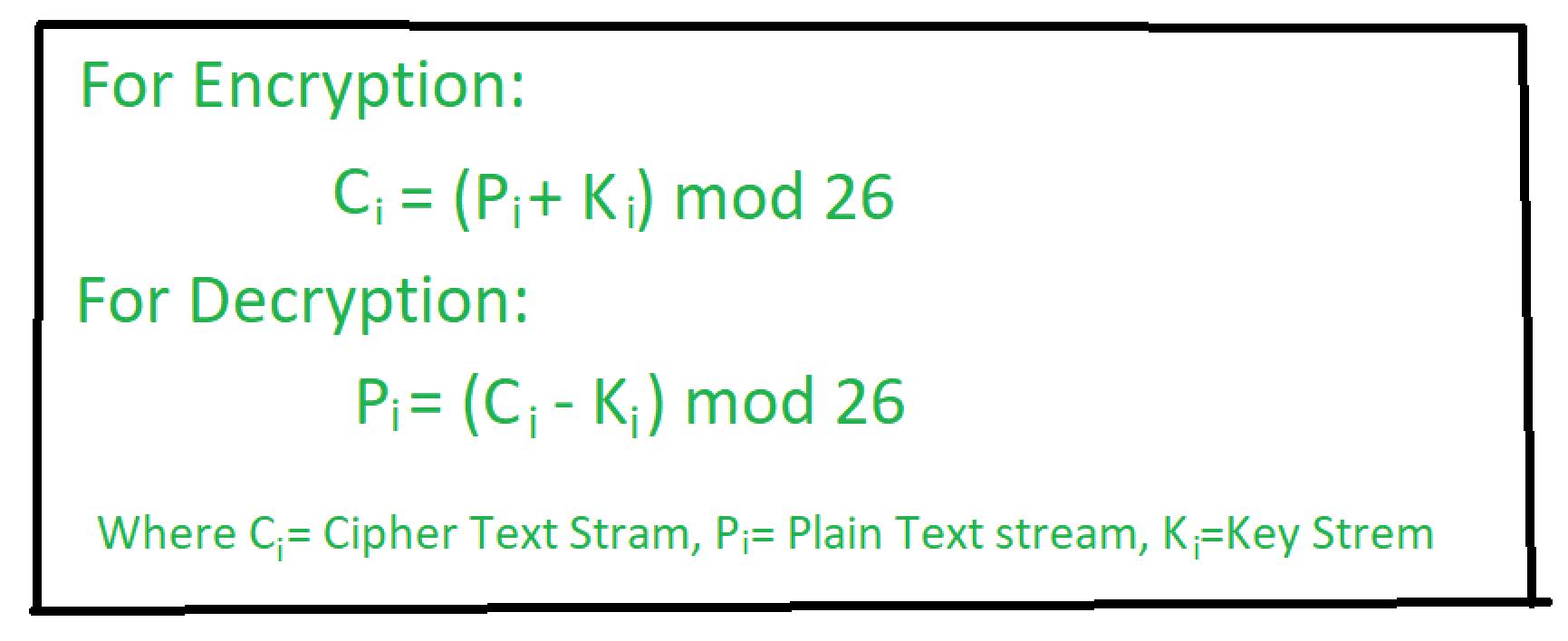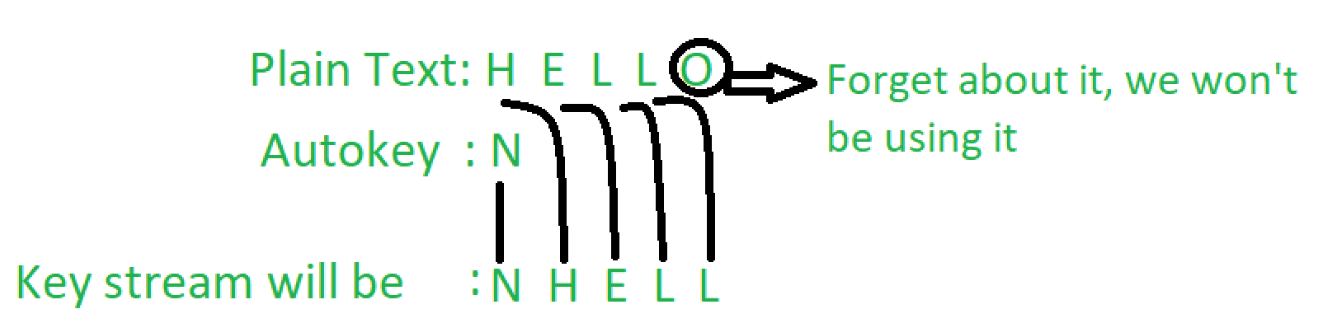# Autokey Cipher | Symmetric Ciphers

Autokey Cipher is a polyalphabetic substitution cipher. It is closely related to the Vigenere cipher but uses a different method of generating the key. It was invented by Blaise de Vigenère in 1586. In general, more secure than the Vigenere cipher.Example-1:

```Plaintext = "HELLO"
Autokey = N
Ciphertext = "ULPWZ" ```

Example-2:

```Plaintext = "GEEKSFORGEEKS"
Autokey = P
Ciphertext = "VKIOCXTFXKIOC" ```

In this cipher, the key is a stream of subkeys which is used to encrypt the corresponding character in the plaintext.As shown, the autokey is added at the first of the subkeys.

```Let's explain Example 1:

Given plain text is : H E L L O
Key is              : N H E L L

Let's encrypt:

Plain Text(P)       : H   E   L   L   O
Corresponding Number: 7   4   11  11  14
Key(K)              : N   H   E   L   L
Corresponding Number: 13  7   4   11  11
---------------------
Applying the formula: 20  11  15  22  25

Corresponding
Letters are         : U    L   P   W   Z

Hence Ciphertext is: ULPWZ

Let's decrypt:

Cipher Text(C)      : U   L   P   W   Z
Key(K)              : N   H   E   L   L
---------------------
Applying the formula: H   E   L   L   O

Hence Plaintext is: HELLO ```

Here’s the java code for Autokey Cipher.

## Java

 `// A JAVA program to illustrate ` `// Autokey Cipher Technique ` ` `  `// Importing required library ` `import` `java.lang.*; ` `import` `java.util.*; ` ` `  `public` `class` `AutoKey { ` ` `  `    ``private` `static` `final` `String alphabet = ``"ABCDEFGHIJKLMNOPQRSTUVWXYZ"``; ` ` `  `    ``public` `static` `void` `main(String[] args) ` `    ``{ ` `        ``String msg = ``"HELLO"``; ` `        ``String key = ``"N"``; ` ` `  `        ``// This if statement is all about java regular expression ` `        ``// [] for range ` `        ``// // Extra \ is used to escape one \ ` `        ``// \\d acts as delimiter ` `        ``// ? once or not at all ` `        ``// . Any character (may or may not match line terminators) ` `        ``if` `(key.matches(``"[-+]?\\d*\\.?\\d+"``)) ` `            ``key = ``""` `+ alphabet.charAt(Integer.parseInt(key)); ` `        ``String enc = autoEncryption(msg, key); ` ` `  `        ``System.out.println(``"Plaintext : "` `+ msg); ` `        ``System.out.println(``"Encrypted : "` `+ enc); ` `        ``System.out.println(``"Decrypted : "` `+ autoDecryption(enc, key)); ` `    ``} ` ` `  `    ``public` `static` `String autoEncryption(String msg, String key) ` `    ``{ ` `        ``int` `len = msg.length(); ` ` `  `        ``// generating the keystream ` `        ``String newKey = key.concat(msg); ` `        ``newKey = newKey.substring(``0``, newKey.length() - key.length()); ` `        ``String encryptMsg = ``""``; ` ` `  `        ``// applying encryption algorithm ` `        ``for` `(``int` `x = ``0``; x < len; x++) { ` `            ``int` `first = alphabet.indexOf(msg.charAt(x)); ` `            ``int` `second = alphabet.indexOf(newKey.charAt(x)); ` `            ``int` `total = (first + second) % ``26``; ` `            ``encryptMsg += alphabet.charAt(total); ` `        ``} ` `        ``return` `encryptMsg; ` `    ``} ` ` `  `    ``public` `static` `String autoDecryption(String msg, String key) ` `    ``{ ` `        ``String currentKey = key; ` `        ``String decryptMsg = ``""``; ` ` `  `        ``// applying decryption algorithm ` `        ``for` `(``int` `x = ``0``; x < msg.length(); x++) { ` `            ``int` `get1 = alphabet.indexOf(msg.charAt(x)); ` `            ``int` `get2 = alphabet.indexOf(currentKey.charAt(x)); ` `            ``int` `total = (get1 - get2) % ``26``; ` `            ``total = (total < ``0``) ? total + ``26` `: total; ` `            ``decryptMsg += alphabet.charAt(total); ` `            ``currentKey += alphabet.charAt(total); ` `        ``} ` `        ``return` `decryptMsg; ` `    ``} ` `} `

```Output:

Plaintext : HELLO
Encrypted : ULPWZ
Decrypted : HELLO

```

My Personal Notes arrow_drop_upTechnical Content Engineer at GeeksForGeeks

If you like GeeksforGeeks and would like to contribute, you can also write an article using contribute.geeksforgeeks.org or mail your article to contribute@geeksforgeeks.org. See your article appearing on the GeeksforGeeks main page and help other Geeks.

Please Improve this article if you find anything incorrect by clicking on the "Improve Article" button below.

Article Tags :
Practice Tags :

Be the First to upvote.

Please write to us at contribute@geeksforgeeks.org to report any issue with the above content.